# Difference between revisions of "De Moivre formula"

The formula expressing the rule for raising a complex number, expressed in trigonometric form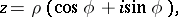to an-th power. According to de Moivre's formula the modulusof the complex number is raised to that power and the argumentis multiplied by the exponent: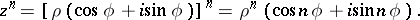The formula was found by A. de Moivre (1707), its modern notation was suggested by L. Euler (1748).

De Moivre's formula can be used to express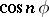and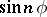in powers of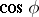and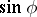: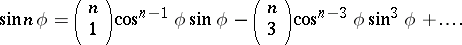Inversion of de Moivre's formula leads to a formula for extracting roots of a complex number: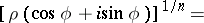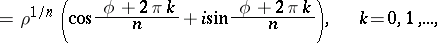which is also sometimes called de Moivre's formula.